Function Repository Resource:

# NestedBranching

Generate a nested branching model

Contributed by: Katja Della Libera
 ResourceFunction["NestedBranching"][b,n] generates a branching model of depth n specified by the rule b, a list of complex numbers representing the result of the first step.

## Details and Options

The "stem" of the model is assumed to be a line from -1 to 0.
The option "Output" defaults to "Graphic", which can be used to determine the output format and accepts the following specifications:
 "Graphic" a visual representation of the model turned by 90° to face upward "Points" a nested list representing the points at each layer "EndPoints" the endpoints of the model "Lines" a list of lists of length two representing the lines with no rotation or conversion from complex numbers

## Examples

### Basic Examples (1)

Get six layers of a branching model with two branches:

 In:=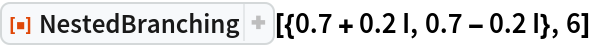Out=### Scope (2)

Retrieve the points instead of a graphic:

 In:=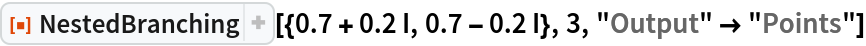Out=Visualize the points using ComplexListPlot:

 In:=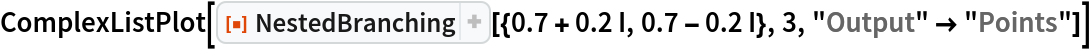Out=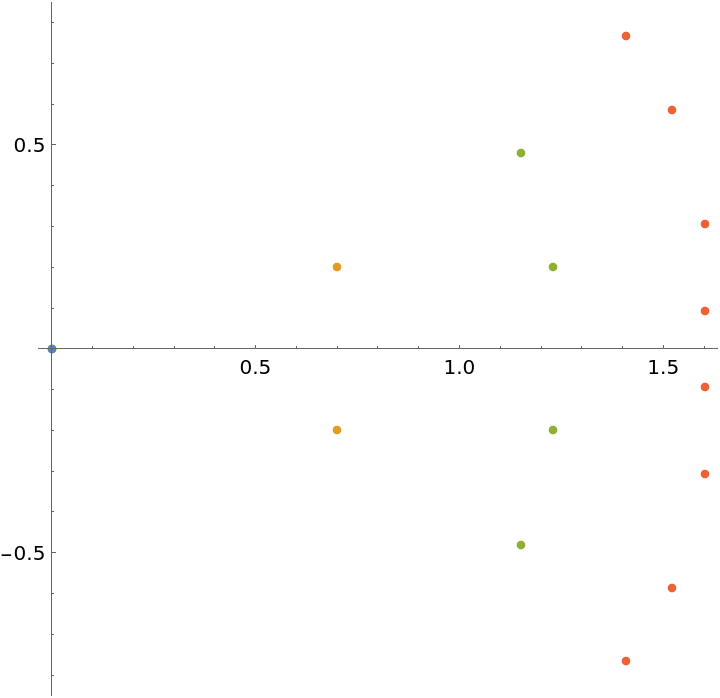The endpoints are just the last list in the points:

 In:=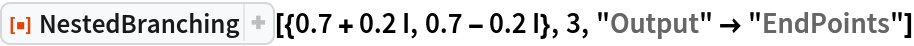Out=The lines can also be retrieved:

 In:=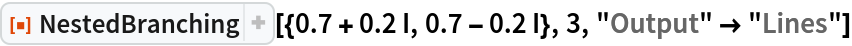Out=The model works for any number of branches and does not have to be symmetric:

 In:=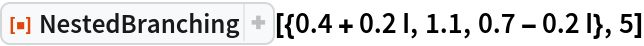Out=### Neat Examples (1)

A simple Manipulate allows for the manual manipulation of the real and imaginary parts of each value in b:

 In:=Out=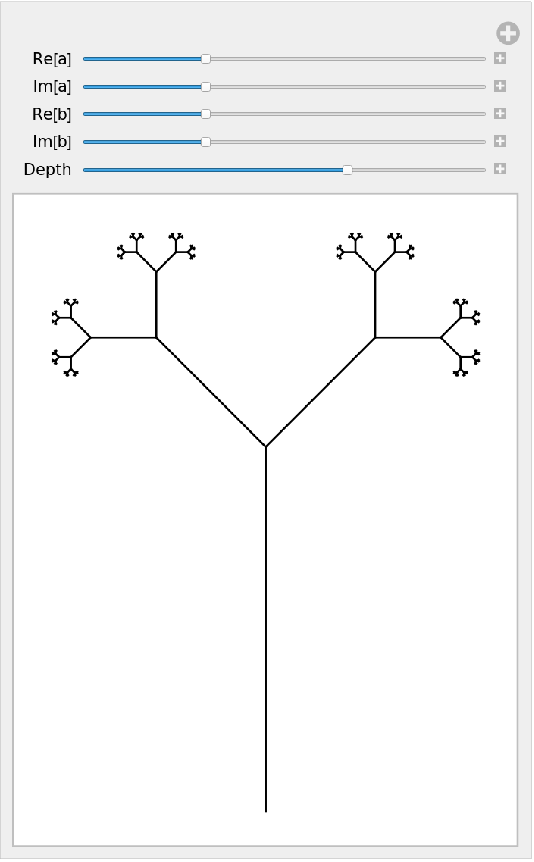## Publisher

Katja Della Libera

## Version History

• 1.0.0 – 17 June 2020